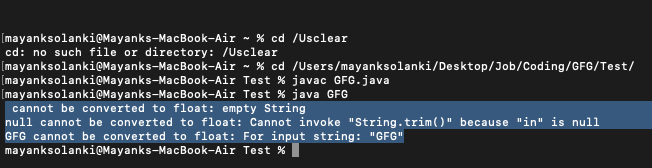Java Program to Convert String to Float Value

• Last Updated : 05 Aug, 2021

Given a string “str” in Java, the task is to convert this string to float type.

Methods:

This can be done by two methods as listed below:

1. Using parseFloat() Method
2. Using valueOf() Method

Let us discuss above two ways of implementing the methods to get a fair understanding of the conversion of string to float values.

Method 1: Using parseFloat()

The parseFloat() method in Float Class is a built-in method in Java that returns a new float initialized to the value represented by the specified String, as done by the valueOf method of class Float.

Syntax:

public static float parseFloat(String s) ;

Parameters: It accepts a single mandatory parameter s which specifies the string to be parsed.

Return type: It returns e float value represented by the string argument.

Exceptions:

• NullPointerException: When the string parsed is null
• NumberFormatException: When the string parsed does not contain a parsable float

Example 1

Java

 // Java Program to Convert String to Float Value by // Implementing parseFloat() method of Float class   // Importing input output classes import java.io.*;   // Main class class GFG {       // Main driver method     public static void main(String[] args)     {         // Input string         String str = "100";           // Returning the float value         // represented by the string argument         float val = Float.parseFloat(str);           // Prints the float value of the string         System.out.println("String is converted to float "                            + val);     } }

Output

String is converted to float 100.0

Example 2: To show unsuccessful conversion

Java

 // Java Program to Convert String to Float Value by // Implementing parseFloat() method of Float class // Where Exception Message is thrown   // Importing input output classes import java.io.*;   // Main class class GFG {      // Method 1    // To convert string to float    public static void convertStringToFloat(String str) {         float floatValue;         // Try block to check for exceptions       try {            // Convert string to float          // using parseFloat() method          floatValue = Float.parseFloat(str);            // Print the expected float value          System.out.println(             str             + " after converting into float = "             + floatValue);       }         // Catch block to handle the exception       catch (Exception e) {            // Print the exception message          // using getMessage() method          System.out.println(             str             + " cannot be converted to float: "             + e.getMessage());       }    }      // Method 2    // Main driver code    public static void main(String[] args) {         // Declaring and initializing custom strings values       String str1 = "";       String str2 = null;       String str3 = "GFG";         // Convert string to float       // using parseFloat() method       convertStringToFloat(str1);       convertStringToFloat(str2);       convertStringToFloat(str3);    } }

Output:Method 2: Using valueof() method

The valueOf() method of the Float class converts data from its internal form into human-readable form. The valueOf() method converts data from its internal form into a human-readable form. It is a static method that is overloaded within a string for all of Java’s built-in types so that each type can be converted properly into a string.

It is called when a string representation of some other type data is needed-for example during concatenation operation.you can call this method with any data type and get a reasonable String representation valueOf() returns java.lang.Integer, which is the object representative of the integer Few forms of valueOf()

Syntax:

Float.valueOf(str);

Returns:

• It returns string representation of given value
• valueOf(iNum); // Returns the string representation of int iNum.
• String.valueOf(sta); // Returns the string representation of the boolean argument.
• String.valueOf(fNum); // Returns the string representation of the float fnum.
• String.valueOf(data, 0, 15); // Returns the string representation of a specific subarray of the chararray argument.
• String.valueOf(data, 0, 5); // Returns the string of charArray 0 to 5
• String.valueOf(data, 7, 9); // Returns the string of charArray starting index 7 and total count from 7 is 9.

Example

Java

 // Java Program to Convert String to Float Value // Using valuesOf() method   // Importing input output classes import java.io.*;   // Main class class GFG {       // Method 1     // To convert String to Float     public static float convertStringToFloat(String str)     {         // Convert string to float         // using valueOf() method         return Float.valueOf(str);     }       // Method 2     // Main driver method     public static void main(String[] args)     {           // Custom input string value         String stringValue = "1.0";           // Expected float value         float floatValue;           // Converting string to float         floatValue = convertStringToFloat(stringValue);           // Printing  the expected float value         System.out.println(             stringValue + " after converting into float = "             + floatValue);     } }

Output

1.0 after converting into float = 1.0

Attention reader! Don’t stop learning now. Get hold of all the important Java Foundation and Collections concepts with the Fundamentals of Java and Java Collections Course at a student-friendly price and become industry ready. To complete your preparation from learning a language to DS Algo and many more,  please refer Complete Interview Preparation Course.

My Personal Notes arrow_drop_up
Recommended Articles
Page :